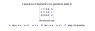# Matrices-back substitution

• TyErd

## Homework Statement

I have attached the question

## The Attempt at a Solution

I think the answer is no solution because there is 5 variables but only 3 equations. Is that correct?

#### Attachments

•zzzzzzzzzzzzzz.png
3.7 KB · Views: 482
TyErd said:

## Homework Statement

I have attached the question

## The Attempt at a Solution

I think the answer is no solution because there is 5 variables but only 3 equations. Is that correct?

No, that is not the reason. Look at the final row; it is shorthand for an equation involving x1, x2, x3, x4, x5. What is the equation?

RGV

do you mean 0x1 + 0x2 + 0x3 + 0x4 + 0x5 = 4?

if the third is also an equation that means there must be an answer right? So because we have to assign variables and we have 5unknowns and 3 equations that must mean two values will be variables right?? so the answer has to be B yeah??

TyErd said:
do you mean 0x1 + 0x2 + 0x3 + 0x4 + 0x5 = 4?
For what values of the variables x1, x1, x2, x3, and x4 will this be a true statement?

TyErd said:
if the third is also an equation that means there must be an answer right?
Not necessarily. There are three possibilities for a system of equations (which are here represented by an augmented matrix):
1) a unique solution
2) multiple solutions
3) no solution.
TyErd said:
So because we have to assign variables and we have 5unknowns and 3 equations that must mean two values will be variables right?? so the answer has to be B yeah??